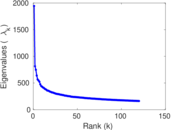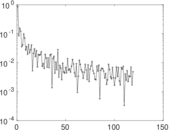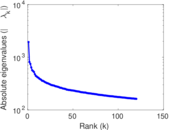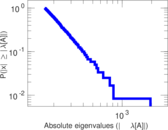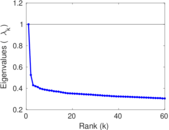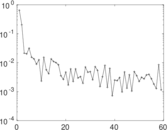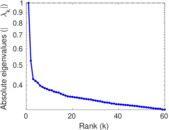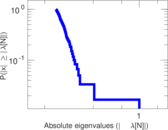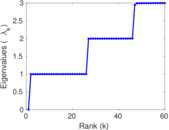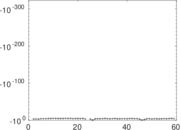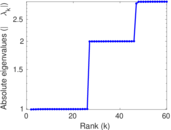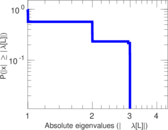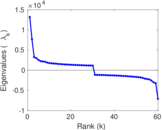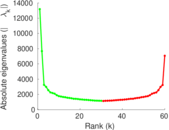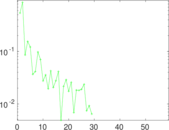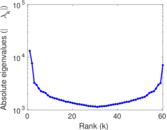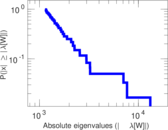# NIPS full papers

This is the bipartite document–word dataset of NIPS full papers. Left nodes are documents and right nodes are words. Edge weights are multiplicities.

 Code `NI` Internal name `bag-nips` Name NIPS full papers Data source http://archive.ics.uci.edu/ml/datasets/Bag+of+Words AvailabilityDataset is available for download Consistency checkDataset passed all tests Category Text network Node meaning Document, word Edge meaning Occurrence Network formatBipartite, undirected Edge typeUnweighted, multiple edges

## Statistics

 Size n = 13,875 Left size n1 = 1,500 Right size n2 = 12,375 Volume m = 1,932,365 Unique edge count m̿ = 746,316 Wedge count s = 316,414,350 Claw count z = 60,669,295,416 Cross count x = 10,271,177,488,043 Square count q = 7,325,274,840 4-Tour count T4 = 59,870,386,232 Maximum degree dmax = 1,455 Maximum left degree d1max = 914 Maximum right degree d2max = 1,455 Average degree d = 278.539 Average left degree d1 = 1,288.24 Average right degree d2 = 156.151 Fill p = 0.040 205 6 Average edge multiplicity m̃ = 2.589 20 Size of LCC N = 13,875 Diameter δ = 6 50-Percentile effective diameter δ0.5 = 3.028 37 90-Percentile effective diameter δ0.9 = 3.805 67 Median distance δM = 4 Mean distance δm = 3.219 19 Gini coefficient G = 0.821 596 Balanced inequality ratio P = 0.140 265 Left balanced inequality ratio P1 = 0.454 947 Right balanced inequality ratio P2 = 0.178 579 Relative edge distribution entropy Her = 0.892 567 Power law exponent γ = 1.300 92 Tail power law exponent γt = 1.521 00 Degree assortativity ρ = −0.042 518 0 Degree assortativity p-value pρ = 1.267 82 × 10−295 Spectral norm α = 1,943.24 Algebraic connectivity a = 0.996 481 Spectral separation |λ1[A] / λ2[A]| = 2.387 05 Controllability C = 10,875 Relative controllability Cr = 0.783 784

## Plots

### Fruchterman–Reingold graph drawing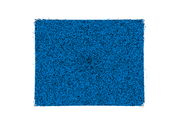### Degree distribution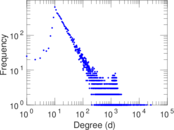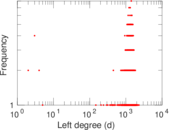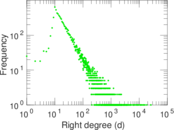### Cumulative degree distribution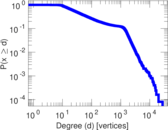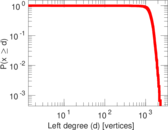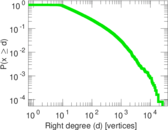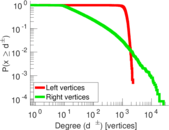### Lorenz curve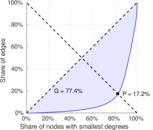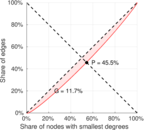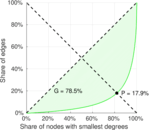### Spectral distribution of the adjacency matrix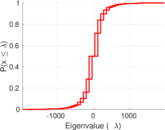### Spectral distribution of the normalized adjacency matrix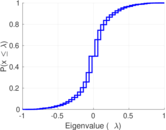### Spectral distribution of the Laplacian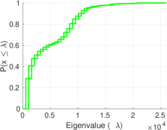### Spectral graph drawing based on the adjacency matrix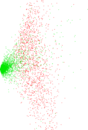### Spectral graph drawing based on the Laplacian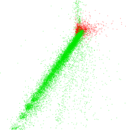### Spectral graph drawing based on the normalized adjacency matrix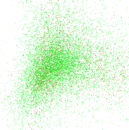### Degree assortativity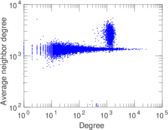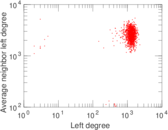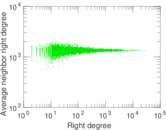### Zipf plot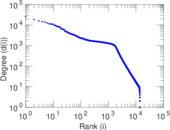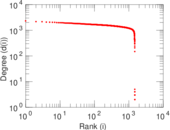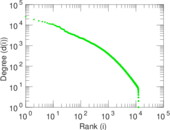### Hop distribution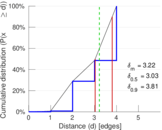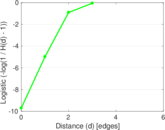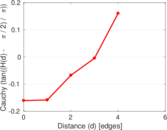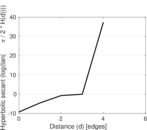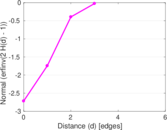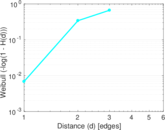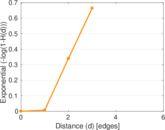### Double Laplacian graph drawing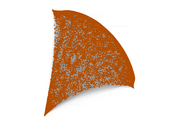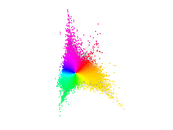### Delaunay graph drawing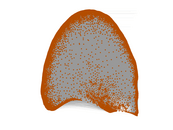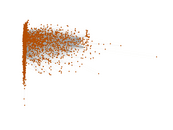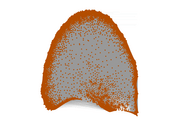### Edge weight/multiplicity distribution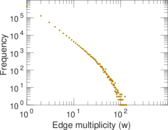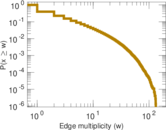### Matrix decompositions plots